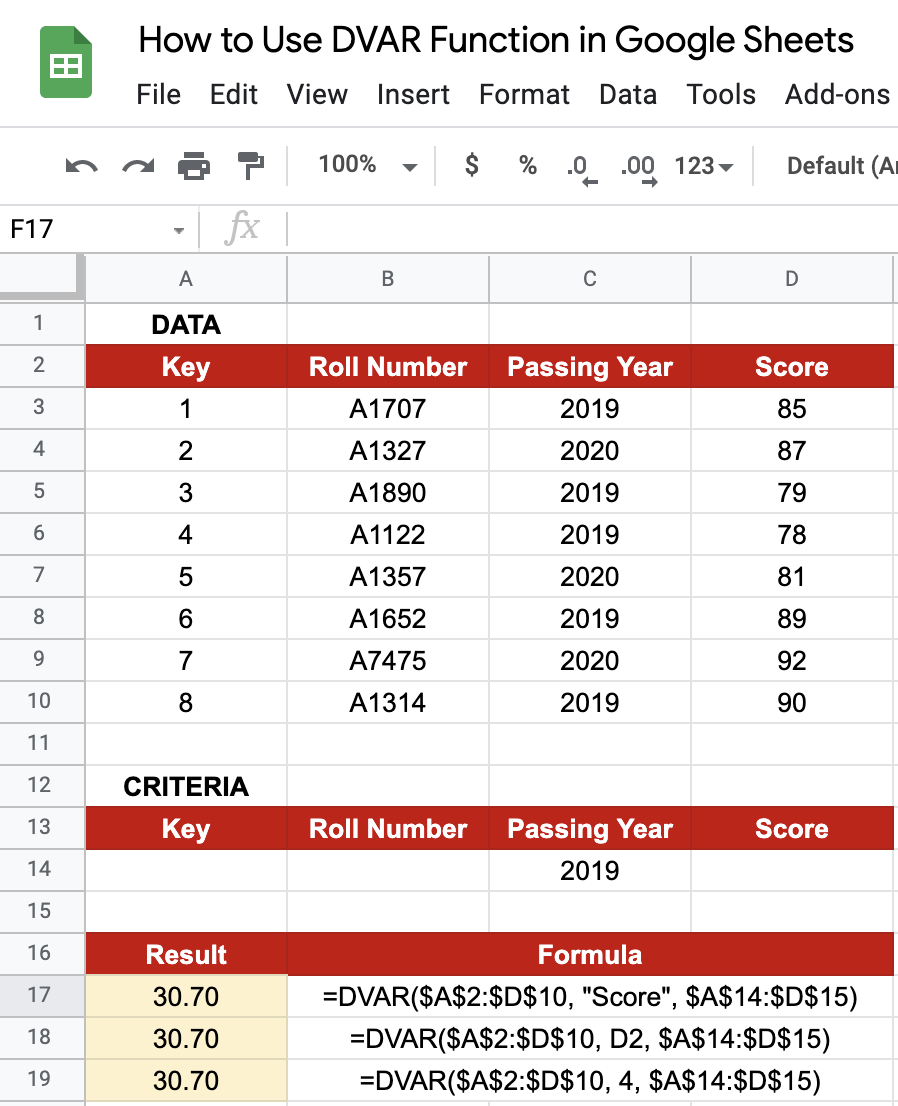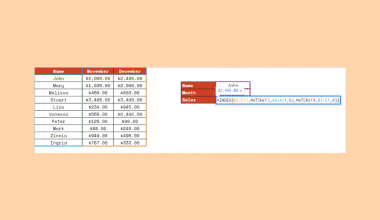How to Use DVAR Function in Google Sheets

The DVAR function in Google Sheets is useful if you need to estimate the variance of a population, based on a sample by using the numbers in a field (column) of records in a list or database that matches the conditions that you specify.

The DVAR() function is a built-in function that is categorized as a Database Function. It can be used as a worksheet function and can be entered as part of a formula in a cell of a worksheet.

For all practical purposes, the application of the DVAR() function is pretty much the same as that of the DVARP() function, except that here we are estimating the population variance from a sample data, whereas in DVARP() we are calculating the variance from the entire population of numbers. You can find our article on the DVARP function here.

What is a variance, though?

For a given data, the variance, a measure of dispersion, measures variability from the average or mean. In simple terms, it is a measure of how much a given value (or data point) varies from the central tendency of the group.

Let’s take an example.

I’d like to investigate the performance of students in mathematics examinations across my city’s schools.

I float an online survey and record the responses from students across different schools. Since I do not have responses from every single student, I will have to estimate the parameters from the sample of records I have. I can make a first approximation based on the responses recorded and try to guess, but I still have to discount the accuracy somewhat based on the smaller sample size.

This is where the DVAR() function will help me. From the variance thus calculated, I will get a deviation from the standard calculated solution that I can expect in reality, on the date of the vaccination. It tells me how reliable my prediction is, based on the survey results.

That’s just one example. There are plenty of other use-cases for this function in real life. Great! Let’s dive right into such real-business use-cases, where we will deal with actual values and as well as learn how we can write our own DVAR() function in Google Sheets to calculate variances in data.

The Anatomy of the DVAR Function

So the syntax (the way we write) of the DVAR function is as follows:

=DVAR(database, field, criteria)

Let’s dissect this thing and understand what each of the terms means:

• = the equal sign is just how we start any function in Google Sheets.
• DVAR() is our DVAR function. DVAR will return the variance of an entire population based on a sample selected from a database table-like array or range using a SQL-like query.
• database denotes an array or range containing the data to consider, structured in such a way that the first row contains the labels for each column’s values
• field indicates the column in the database which contains the values to be extracted and operated on.
• field may either be a text label corresponding to a column header in the first row of the database or a numeric index indicating which column to consider, where the first column has a value = 1.
• criteria is an array or range containing zero or more criteria to filter the database values before operating.

For better understanding of the difference between estimating variance from a population vs. a sample, we shall be explaining the function using the same examples from our guide on DVARP() function, so that you can compare the two.

A Real Example of Using DVAR Function

Take a look at the example below to see how DVAR() functions are used in Google Sheets.The above figures represent the marks earned by students in a given class over the course of two years, 2019 and 2020, in Mathematics. The difference here is that this is not the exhaustive set of students, and is a sample. The objective though, still is to find the dispersion in the marks from the average marks secured across all the students, for a given year.

Just below the captured data, I have given a provision to enter the criteria based on which the data will be filtered. This is not a required criterion, and the function will still function properly if we leave it blank. As you can see below, I have obtained the variance for scores obtained in the year 2019.I have shown a couple of different ways to input the field, all of which give the same result. Note that the variance when we consider this as a sample has increased to 30.70 from 24.56 (when considered as the entire population).

Why is this value higher?

When we calculate variance based on the entire population, the denominator of the formula is the total number of items. However, when the variance is calculated from sample data, we subtract a value of 1 before dividing the sum of the squared deviations. For this very reason, the value of variance calculated from sample data is higher than the value that could have been found out by using population data. The logic of doing so is to compensate for our lack of information about the population data. When sample data is taken for most of the statistical purposes, it is accompanied by a lack of information about the majority of data. In order to compensate for this, the value of variance and standard deviation, which is the square root of the variance, are higher in case of sample data than variance from population data.

You may try changing the criteria and see how the result changes. Go ahead and make a copy of the spreadsheet using the link I have attached below:

Awesome! Let’s begin our DVAR() function in Google Sheets.

How to Use DVAR Function in Google Sheets

1. Let’s see how to write your own DVAR() function, step-by-step. First of all, I have pasted sales data from a single retail store across three years, from 2018 to 2020, for three apparel brands – Nike, Adidas, and Puma.1. Now, simply click on any cell to make it the active cell. For this guide, I will be selecting F14, where I want to show my results.
2. Next, simply type the equal sign ‘=‘ to begin the function and then followed by the name of the function, which is our ‘dvarp‘ (or DVARP, whichever works).
3. You should find that the auto-suggest box appears with our function of interest. Continue by entering the first opening bracket ‘(‘. If you get a huge box with text in it, simply hit the arrow in the top-right hand corner of the box to minimize it. You should now see it as follows:1. Now, the fun begins! Let’s give the required inputs to the function to get the variance in unit sales, per the filtration criteria we have given above the data:1. Take note of how I’ve specified conditions to limit the data to the Nike brand (selecting only the years 2019 and 2020). The criteria for the formula are input as A1:F3 to account for all the criteria mentioned if any.
2. Once you’ve entered the necessary database, field, and criterion values, or you’ve done what I did, make sure to close the brackets , as shown below.1. Finally, just hit your Enter key. If you followed my instructions, you should have gotten 75,000 as the function’s output, which is nothing more than the variance of Nike and Puma combined unit sales (for the criteria specified above the data table).As explained in the previous example, note that the variance is higher here since we are considering the data to be a sample and not the entire population.

That’s pretty much it. You have everything you need to get started with the DVAR function on Google Sheets. I recommend experimenting with the DVAR function, combining it with the numerous Google Sheets formulas available, and seeing what you can come up with. 🙂Our goal this year is to create lots of rich, bite-sized tutorials for Google Sheets users like you. If you liked this one, you'll love what we are working on! Readers receive ✨ early access ✨ to new content.

You May Also LikeHow to Use DELTA Function in Google Sheets

The DELTA function in Google Sheets is used to compare two numeric values, and returns the value ‘1’…How to Use the RATE Function in Google Sheets

The RATE function in Google Sheets is used to calculate the interest rate of an annuity investment based…How To Use INDEX and MATCH Together in Google Sheets

The INDEX and MATCH together in Google Sheets come useful if you want to perform lookups. Using the…How to Generate Random Numbers in Google Sheets

Learning how to generate random numbers in Google Sheets is useful to create a unique number that does…